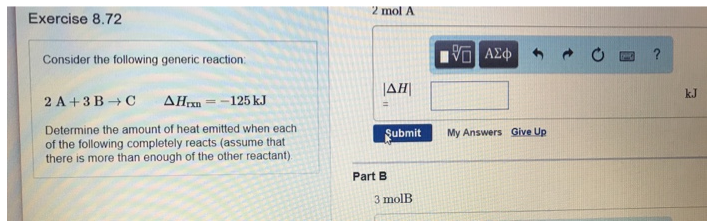# Problem: Consider the following generic reaction: 2A + 3B → C       ΔHrxn = -125 kJ Determine the amount of heat emitted when each of the following completely reacts (assume that there is more than enough of the other reactant)Part A2 mol APart B3 mol B

###### FREE Expert Solution
98% (93 ratings)###### Problem Details

Consider the following generic reaction:

2A + 3B → C       ΔHrxn = -125 kJ

Determine the amount of heat emitted when each of the following completely reacts (assume that there is more than enough of the other reactant)

Part A

2 mol A

Part B

3 mol B